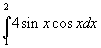# Definite Integral question

b_roberts

## Homework Statement

The letter W is defined as. The value of W, correct to the nearest hundredth, is?

## The Attempt at a Solution

Now, I had this question on a test and got it wrong. I get 0.93, which I think is correct. Apparently the answer is 0.24. I call BS on my teacher.

Homework Helper
Gold Member
Now, I had this question on a test and got it wrong. I get 0.93, which I think is correct. Apparently the answer is 0.24. I call BS on my teacher.

Well, you're incorrect, as any calculus capable calculator will confirm. So, instead of directing profanities towards your teacher, perhaps you should post your calculations so we can point out where your error(s) are.

b_roberts
How am I wrong? The function is negative from pi/2 to 2 and the area below the x-axis has to be calculated separately as an absolute value and added to the area above the x-axis. Of course an integral calculator will get the question wrong, they can't handle area below the x-axis.

l'Hôpital
How am I wrong? The function is negative from pi/2 to 2 and the area below the x-axis has to be calculated separately as an absolute value and added to the area above the x-axis. Of course an integral calculator will get the question wrong, they can't handle area below the x-axis.

Who said anything about the area under a curve? It says compute a definite integral, not "Find the area under the curve."

b_roberts
The definite integral is the area under the curve...

l'Hôpital
^
That's a common misconception. The definite integral can get you the area under the curve, but that doesn't mean it is.

b_roberts
So what you're saying is that if it doesn't ask for me to compute the area for a definite integral, that I should just calculate it straight away and disregard if it is negative?

l'Hôpital
Yes.

Remember the fundamental theorem of Calculus:

For a sufficiently nice function,

$$\int_a^b f(x) dx = F(b) - F(a)$$

Where a and b are the bounds and F is the anti-derivative.

It doesn't care about negative or positive. All it cares about is the value of F at b and a.

b_roberts
Alright. That makes sense. Thank you.

union68
The definite integral is the signed, net area under the curve. That's quite easy to forget.

That's why

$$\int_0^{2\pi} \cos x \ dx$$

evaluates to zero. There is the same amount of area above the x-axis as there is below it.

Homework Helper
Gold Member
The definite integral is the area under the curve...

That's not the definition of a definite integral. When in doubt, you should always turn to the definitions.In this case, the definition involves a limit of a summation. Thinking of a definite integral as being an area under a curve is only strictly true when the curve is above the x-axis over the entire interval.

If you were asked to find the area between the curve $f(x)=4\sin x\cos x$ and the x-axis on the interval $x\in [1,2]$, then your method would be correct. However, that wasn't what you were asked.